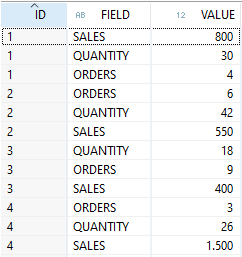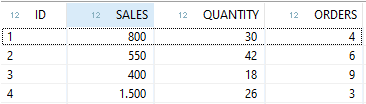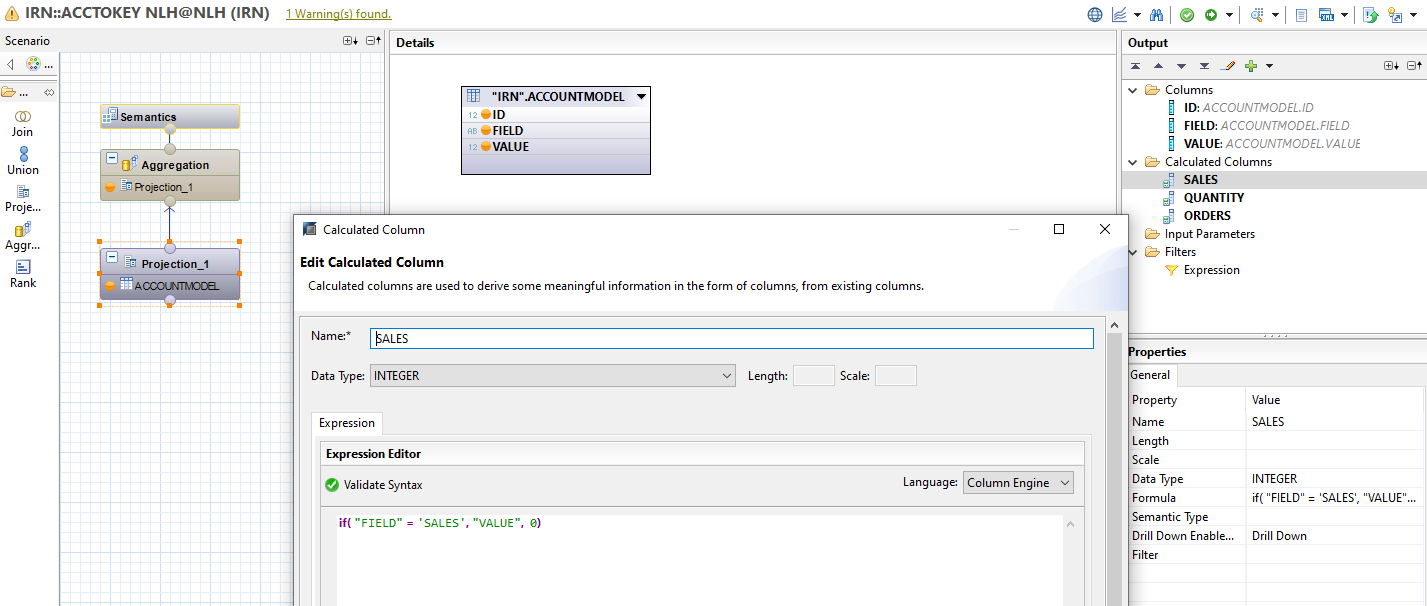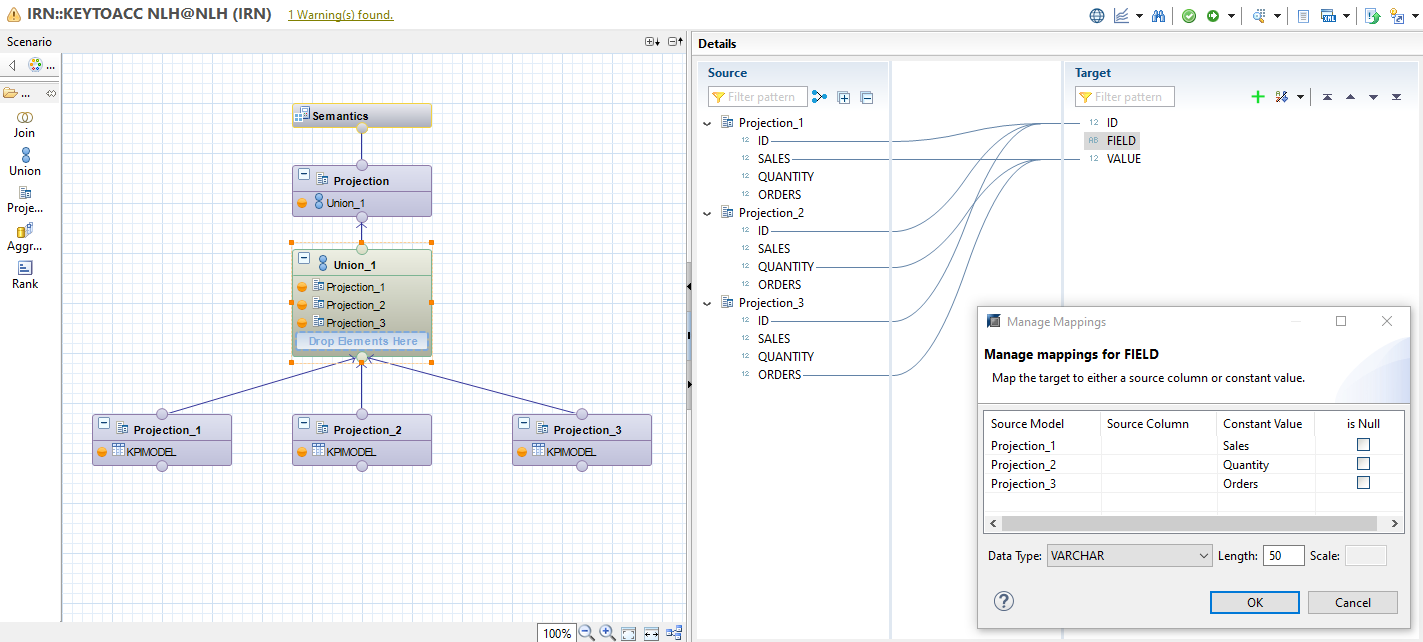# Handling account & key figure model in SAP HANA SQLScript & CalcViewWritten By: Irvin - 03 February 2022
(updated on: 20 September 2022)

In this blog post, we look at several variants for transforming or pivoting/unpivoting a dataset between the account model and the key figure model. For this purpose, we will first illustrate and explain the terms of the account model and key figure model, respectively, and then show the possibilities for transformation that SAP HANA offers.

The inclined SAP Business Warehouse (BW) user will already be familiar with the terms account model and key figure model.Account Model

The table above visualizes a data modeling according to the account model.

It contains a column with the name of the key figure and a second column with the corresponding value. Regardless of the number of key figures, only two columns are used here.Key Figure Model

The table above, on the other hand, shows the key figure model.

Thus, a separate column is modeled for each key figure. The name of the key figure is also the name of the column and the values of the column contain the respective values of the key figure.

If there is no value for the key figure of a data record, it is filled with a default value, e.g. null or 0.

There are a number of advantages and disadvantages resulting from these approaches:

• No unnecessary values are saved in the account model (null or 0 in the key figure model).
• A new key figure can be modeled in the account model without changing the data model.
• The key figure model facilitates the analysis and is therefore preferably used in the frontend/reporting - therefore a transformation from account model to key figure model may have to be done in the backend
• For row-based tables, the performance of the account model is stronger

Historically, the use of both of these models was essential, as modeling according to the account model enabled better performance of the BW system, but the key figure model was (and still is) essential for reporting. With the introduction of the column-based architecture of the tables in the SAP HANA database, the use of the account model is no longer performance-relevant, but may still be justified for architectural reasons.

### From account model to key figure model (pivot)

Now let's look at a scenario where we want to transform a data model based on the account principle into the key figure model in the HANA context. Architecturally, we have the choice of enabling this transformation either graphically in a Calculation View, or implementing it as part of a script-based HANA object, such as a Table Function, using SQLScript.

In the SQLScript-based approach, a CASE-WHEN statement is used for each key figure to be modeled:

`-- Account Model to Key Figure ModelSELECT     ID,     SUM (CASE           WHEN "FIELD" = 'SALES'           THEN "VALUE"           ELSE 0     END) as "SALES",     SUM (CASE           WHEN "FIELD" = 'QUANTITY'           THEN "VALUE"           ELSE 0     END) as "QUANTITY",     SUM (CASE           WHEN "FIELD" = 'ORDERS'           THEN "VALUE"           ELSE 0     END) as "ORDERS"FROM    "IRN"."ACCOUNTMODEL"GROUP BY    IDORDER BY    ID;`

Since apart from the CASE-WHEN framework no extraordinary SQL logic is required here, the same functionality can also be implemented using a Calculation View. Calculated Columns are used for this purpose. Each CASE-WHEN statement is equivalent to a Calculated Column:Pivot with Calculation View

### From key figure model to account model (Unpivot)

Here as well, we can use either a Calculation View or SQLScript.

For the graphical solution, we first create a Projection Node for each key figure and then connect it to a Union Node. Afterwards we edit the Union Node by:

• Removing all mappings of the key figures that may already have been entered automatically;
• Using the "+" sign in the "Target" area of the Union Node we create two custom target fields - one for the field names and one for the field values;
• Right click on the custom field for the field labels => Manage Mappings and map the projection nodes with one constant value each to the field for the field labels;
• Map one key figure from each projection node to the field for the field values (according to the previously defined Constant Value to projection node pairs).Unpivot with Calculation View

Analogously, this principle can also be applied via SQLScript. A subselect is written for each key figure and these subselects are connected by UNION ALL:

`-- UNION ALL: Key Figure Model to Account ModelSELECT     ID, 'SALES' as "FIELD", "SALES" as "VALUE"FROM "IRN"."KPIMODEL"     UNION ALLSELECT     ID, 'QUANTITY' as "FIELD", "QUANTITY" as "VALUE"FROM "IRN"."KPIMODEL"     UNION ALLSELECT     ID, 'ORDERS' as "FIELD", "ORDERS" as "VALUE"FROM "IRN"."KPIMODEL";`

Alternatively, HANA also offers the possibility of combining two built-in functions - MAP() and SERIES_GENERATE_INTEGER(). The latter automatically generates a table containing a series of numbers - for us the generated column "ELEMENT_NUMBER" is interesting. The MAP() function allows us to assign a series of initial values to a target value each. So we generate a number series using SERIES_GENERATE_INTEGER() that corresponds to the number of our key figures and form a cross join with the table that contains our key figure based data model. We then use MAP() to correctly populate the two fields for the field label and field value in the SELECT.

`-- MAP(): Key Figure Model to Account ModelSELECT     ID,     MAP(element_number,           1, 'SALES',           2, 'QUANTITY',           3, 'ORDERS')     as "FIELD",     MAP(element_number,           1, "SALES",           2, "QUANTITY",           3, "ORDERS")     as "VALUE"FROM "IRN"."KPIMODEL"CROSS JOIN SERIES_GENERATE_INTEGER(1,1,4);`

Do you have questions about this or other topics? Are you trying to build up the necessary know-how in your department or do you need support with a specific question? We will be happy to help you. Request a non-binding consulting offer today.Topics: SAP HANA SQL